Mechanics

# MUV time diagram

When a body changes its velocity in a constant way, we say that it is in uniformly varied motion (MUV).

Uniformly varied motion (MUV) hourly diagrams are graphs that relate quantities, such as position , velocity , and acceleration, with the passage of time. Using the MUV diagrams , we can calculate the speed of the mobile, its displacement and even its speed variations .

## Velocity by time (vxt) graph in MUV

The graph of velocity versus time in uniformly varied motion is always an ascending or descending line , since velocity in this type of motion is subject to an acceleration or deceleration of constant magnitude.

When the velocity of a mobile increases as a function of time, it is represented as an ascending line. This motion is called accelerated motion .

The formula used to plot v(t) (velocity versus time) is called the hourly function of velocity. This function is shown below: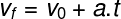Caption :
f – final velocity (m/s)
0 – initial velocity (m/s)
a – average acceleration (m/s²)
t – time interval (s)

The above function is a 1st degree (straight) function. Therefore, for positive values ​​of acceleration, your graph will be an ascending line , as shown below:

If the velocity of the object decreases as a function of time, we say that its motion is retarded In this case, the velocity versus time diagram will also be a straight line, but that line will descend because of the negative acceleration .

In addition, this type of graph is especially useful for calculating the displacement suffered by the mobile. To do this, just calculate the area of ​​this graph.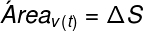In the graph above, although the velocity module is decreasing, it is still located above the abscissa axis (time axis). This indicates that the mobile is still moving away from the origin. It is, therefore, a delayed progressive movement .

## Position graph by time (S xt) in MUV

The position versus time diagram for the MUV is described by the graph of a second degree function called the hourly function of the MUV position . This function can be written in two ways :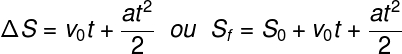Legend:
f – final position (m)
0 – initial position (m)
ΔS = S f – S 0 – displacement (m)
f – final velocity (m/s)
0 – initial velocity (m/s)
a – average acceleration (m/s²)
t – time interval (s)

The position hour function for the MUV indicates that the position (S) versus time (t) graph will have the shape of a parabola. When the speed of the mobile increases as a function of time, we will have a parabola with the concavity facing upwards Watch:

In cases where the motion slows down, we will have a graph of a parabola with the concavity facing downwards Watch:

The most important points of position versus time diagrams are their roots (t’ and t” or t 0 and t f ). These points inform us when the mobile passes through the origin of the reference frame. Furthermore, for the initial time instant (t 0 ), it is possible to determine the initial position (S 0 ) of the mobile: just look at how high the parabola crosses the vertical axis during the initial time instant.

## Graph of acceleration as a function of time (axt) in the MUV

Graphs of acceleration versus time are especially useful in determining whether a body is being accelerated or decelerated. By means of these graphs, it is possible to calculate the variation of the velocity suffered by the mobile (Δv = v f – v 0 ).

For uniformly accelerated motion, we will have a line parallel to the horizontal axis of constant height. Watch:

Since the acceleration line is above the horizontal axis, we say that the motion is accelerated. To determine the change in the speed of the mobile, just calculate the area of ​​this graph.

Don’t stop now… There’s more after the publicity 😉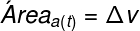When the mobile is undergoing some deceleration , the line a(t) (acceleration as a function of time) will appear below the horizontal axis, since the values ​​of this line will be negative. Watch:

## Summary about the MUV time chart

→ In uniformly accelerated motion , the position versus time graph s(t) will be a parabola with the concavity facing upwards , and its acceleration will be positive.

→ In uniformly decelerated or retarded motion, the position versus time graph s(t) will be a parabola with the concavity facing downwards , and its acceleration will be negative.

→ In the uniformly accelerated motion , the graph of velocity versus time v(t) will be an ascending (rising) line.

→ In uniformly retarded motion , the graph of velocity versus time v(t) will be a descending (going down) line.

→ In uniformly accelerated motion , the graph of acceleration as a function of time a(t) will be a line parallel to the horizontal axis and arranged above it.

→ In uniformly retarded motion , the graph of acceleration as a function of time a(t) will be a line parallel to the horizontal axis and lying below it.

## Solved exercises on MUV graphics

1. The graph of velocity versus time of a mobile moving with constant acceleration is shown below:

Analyze the graph above and answer:

a) What is the initial velocity of the car?

b) What is the final velocity of the car?

c) What is the magnitude of the car’s acceleration?

d) Classify the movement as progressive or regressive, retarded or accelerated.

e) Calculate, through the graph, the magnitude of the displacement suffered by the mobile.

f) Determine at which instant the speed of the mobile will have a magnitude of 60 m/s.

Resolution:

a) Analyzing the graph above, it is possible to notice that, at the initial instant of time (t 0 = 0 s), where the two axes of the graph cross, the speed of the mobile is 20 m/s .

b) The final time instant (t f ) shown in the graph is the instant t = 5 s. At that instant of time, the speed of the mobile is 50 m/s .

c) We can calculate the acceleration of the mobile through the equation of the average acceleration (equivalent to the hourly velocity function):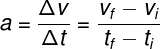Analyzing the graph, it is possible to find the values ​​of f , i , f and i. That way: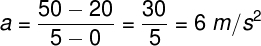The calculation indicates that the speed of the mobile increases, in module, 6 meters per second every second .

d) The velocity line is above the horizontal axis, therefore, the movement is progressive, that is, the mobile moves away from the referential. Also, the line is sloping upward, indicating that its magnitude increases. It is, therefore, an accelerated movement. Therefore, we say that the motion is progressive and accelerated .

e) To calculate the distance traveled by the mobile through the graph, we must calculate the area. The graph has the geometric shape of a trapezoid, whose area is given by the formula: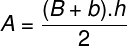Legend:
A
– area of ​​the trapezoid
B – edge of the larger base
b – edge of the smaller base
h – height of the trapeze

We can assimilate the velocity f to the major base B, the initial velocity vi to the minor base b , the final instant of time f to the height h of the trapezoid . With that, we will have: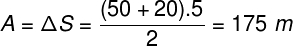f) To determine the speed of the mobile at some time later than those shown in the graph, we use the hourly speed function :As calculated earlier, the acceleration of this mobile is equal to 6 m/s². In order for it to reach a final speed of 60 m/s, we will have the following resolution: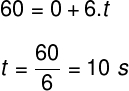Therefore, we can conclude that the mobile will have a speed of 60 m/s at the instant of time t = 10 s .